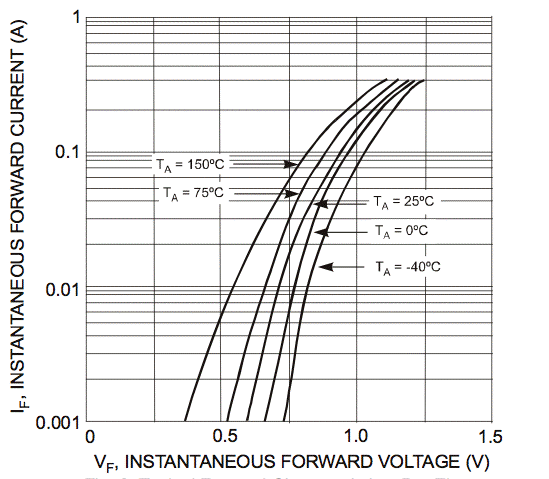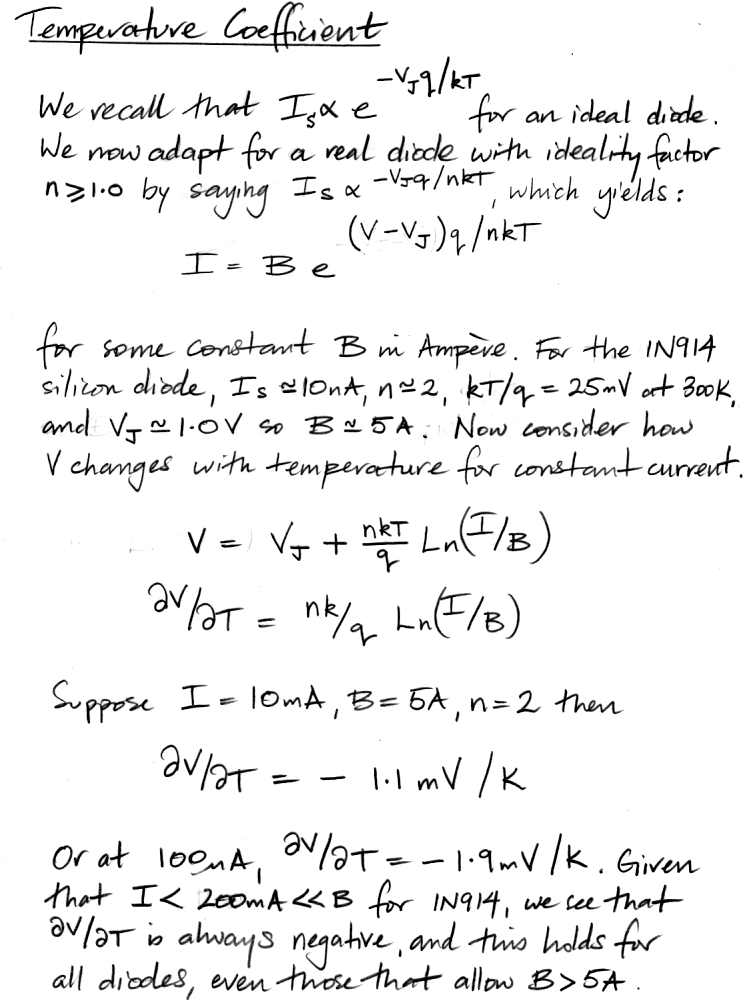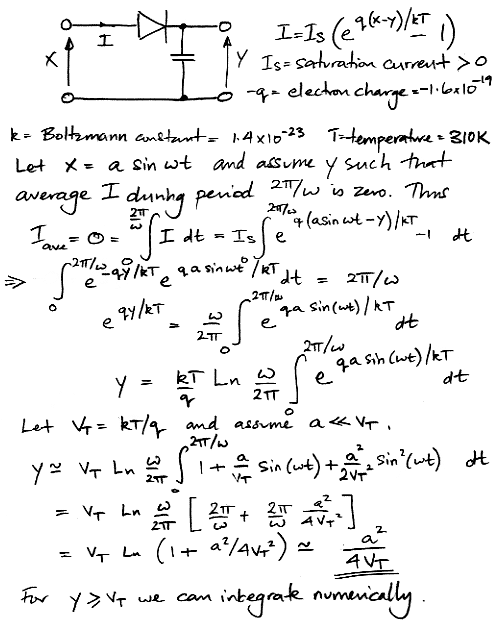# Diodes and Transistors

© 2015-2018 Kevan Hashemi, Brandeis University

## Contents

Introduction
Temperature Coefficient
Diode Power Meter

## Introduction

A diode is a two-port electronic component that allows current to flow more easily in one direction than the other. A transistor is a three-terminal device in which one terminal controls the current flowing through another terminal. The most common diode is the silicon diode, made of a silicon semiconductor junction. Silicon diodes can also be made of germanium, but such devices are now rare. Another common diode is the Schottky diode, made out of a metal-semiconductor junction. The behavior of both all three of these types of diode is described by the Shockley equation, in which the current that flows through the device is exponential with the forward voltage. A bipolar transiostor is made of two silicon junctions back-to-back. We can have PNP or NPN bipolar transistors. The same exponential relationship describes the current flowing through the a bipolar transistor, although in that context the relationship is called the Ebers-Moll equation. In time, we will discuss field effect transistors here as well, but for now we present some details of bipolar transistors.

## Temperature Coefficient

Consider the temperature coefficient of the semiconductor diode's forward voltage drop. This temperature coefficient is negative, meaning that the voltage across a diode or a base-emitter junction for a particular current decreases as we heat up the device. The negative temperature coefficient is the basis of thermal runaway, in which a diode or transistor driven by a fixed voltage gets hotter and hotter and passes more and more current until it over-heats and fails.Figure: Voltage Drop Across BAV99 Diode, Showing Negative Temperature Coefficient.

If we differentiate the Shockley equation with respect to temperature, and assume the saturation current, IS, is constant with temperature, we find that the temperature coefficient of the forward voltage drop must be positive, not negative. But IS increases with temperature also. The following derivation comes from our lecture on diodes.Figure: Forward Voltage Dependence Upon Temperature for a Diode (or for the Base-Emittter Junction of a Bipolar Transistor).

Real silicon diodes don't follow the ideal Shockley equation, but they do follow an exponential relation that we can obtain from the Shockley equation by introducting an ideality factor, n. In our laboratory course, we measure n ≈ 2.0 for the 1N914 and 1N4001 silicon diodes, and 1.0 for the base-emitter junction of the 2N3904 transistor. Assuming an ideality factor 2.0 for the BAV99, and noting V = 0.6 V for I = 1 mA at 25°C in the graph above, we obtain IS = 6 nA. This compares well with the 10-nA reverse current of the BAV99 at 25°C. Looking at the data sheet, we see that for 10 mA, the voltage drop decreases by around 100 mV for a 50°C increase in temperature, which is around −1 mV/°C, as predicted by the above derivation. In this circuit, we use a chain of seven BAV99DW diodes to generate a voltage for a logic power supply. We expect the voltage across this chain to drop by around 7 mV/°C.

## Diode Power Meter

We can use a diode to measure the power of a sinusoidal signal. In amplitude-modulated radio receivers, we can use diodes to convert the amplitude of the radio-frequency signal into a signal representing the modulation envelope. In power-detectors, we use a diode to provide an output that we can use to measure absolute radio-frequency power. The following derivation shows how a diode converts small signal amplitude into a constant voltage.Figure: Conversion of Amplitude into a Constant Voltage by a Diode and Capacitor.

For larger amplitude, we use numerical integration to obtain the voltage on the capacitor. For a comparison of calculated and measured values of the diode output voltage for various amplitudes, see here.